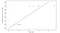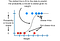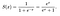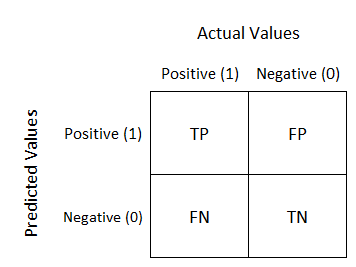Regression technique is not about just about Linear Regression. The power and simplicity of Regression can be used for classification too with the help of Logistic Regression which classifies the output as either 0 or 1 or as True/False (I like to call it Pseudo Classifier as well). So let us continue our journey of ML and dive into Logistic Regression.

1. Why do we need Logistic Regression?

Think about the situation where you have linearity within your data and you have a classification problem then the perfect algorithm in such a situation is Logistic Regression. You may think as the data are linear in nature then why not use Linear Regression instead. You can’t, let us see whyTrying LinearRegression in Impossible case

As you can see from the above case Linear Regression just simply fails. The result will be horrible. We need some other math function which can fit this type of data points. A sigmoid function is a math function having a characteristic “S”-shaped curve or sigmoid curve, which perfectly fit in our case.

This fancy looking formula is the sigmoid function (but don’t worry what we actually use is Logit function, which we’ll discuss later).

So now I believe you have understood that there are cases where we simply can’t use Linear Regression and have to go with Logistic Regression.

2. What is common and what is different between Linear and Logistic Regression?

The most important common thing between both of them is that they both produce continuous output. This two-point will get the idea more clear:

1. In the case of Linear Regression(talking in nutshell), we use OLS (ordinary least square) method to construct Best Fit Line. So to forecast output, we just simply project the point on the x-axis and take its corresponding value on the y-axis.

2. In the case of Logistic Regression(talking in nutshell), we use the Logit function to construct the S-shaped curve. So to classify output, we just simply project the point on the x-axis and if its corresponding value on the y-axis is less than 0.5 is classified as class 0; if more than 0.5 then class 1(default cut off value is 0.5, but can be changed according to needs)Example of Logistic Regression

Note: The Logit function works in such a way that output value will always range in between of 0 to 1.

So the talking points are, they both may be Regression technique but both their use case are absolutely different.

3. Ok so it is a Classification Algorithm, but how it really works? What is the logic as well as maths behind it?

First thing first, let us clear some concepts which are floating around.

1.Sigmoid Function: A sigmoid function is a mathematical function having a characteristic “S”-shaped curve or sigmoid curve. Often, sigmoid function refers to the special case of the logistic function. (But we used modified version ).Sigmoid function

2. Logit Function: If p is a probability, then p/(1 − p) is the corresponding odds; the logit of the probability is the logarithm of the odds, i.e.

( We use this in the algorithm)

3. Sigmoid vs Logit Function: They are nothing more than just reciprocal of each other. Logit function is used in the algorithm but the sigmoid might be useful if you want to transform a real-valued variable into something that represents a probability( to read more in-depth comparison go here).

4. Odds: The ratio of an Event happening to the ration of Event not happening. You can consider Odds as the driving engine behind the algorithm. We use log(odds) (Why? Don’t worry we’ll see).

5. Odds vs Probability: Probability ranges between 0 to 1 whereas Odds can range anything between 0 to ∞. Odds can be considered as a kind of subset of Probability (to read more in-depth comparison go here)

Note: Before moving forward I highly recommend you to watch this video

4. How to build a logistic regression model with Log(odds)?

As I mentioned earlier, log(Odds) of the result are plotted on the S-shaped curve. Now odds can be anything between 0 to ∞. Try to think intuitively for any event which is occurring more frequently vs less frequently. In the 1st case(more frequently), its Odds will be always between 1 to oo (depending upon its frequency) and in the 2nd case(less frequency), Odds will always between 1 to 0. Now this scale is not uniform. So statisticians prefer to take log(odds) which transform them to a uniform scale. Ok, the next thing is how the any Linear data point is actually converted into the log(odds) and can be plotted to the curve. Josh Starmer has clearly explained ‘behind the scene’ of this algorithm. So I highly suggest you see this video. (You may survive without understanding this but don’t be that guy)

6. What about performance metrics? Why it is so essential?

The most important aspect of any algorithm is its performance. The same case goes with this algorithm. As it comes under Classification, we can use all the Classification Performance metrics like Confusion matrix, Precision & Recall, f1-score, AUC & ROC curve, not forget the evergreen sklearn.metrics.accuracy_score. Let us figure out some of them in a nutshell.

i) Confusion Matrix: Powerfull and alone can do the work done.Confusion Matrix

True Positives (TP): These are predicted yes and actually yes
True Negatives (TN): We predicted no, and actually no
False Positives (FP): We predicted yes, but was actually no.
False Negatives (FN): We predicted no, but was yes.

(For more in-depth visit here)

ii) Precision & Recall: A very important application of Confusion matrix.

Precision (true positives / predicted positives) = TP / TP + FP

Sensitivity aka Recall (true positives / all actual positives) = TP / TP + FN

(Please read this blog which is beautifuly explained with story)

iii) ROC & AUC curve: Graph can prove vital too. The area under ROC Curve (or AUC for short) is a performance metric for binary classification problems.

• The AUC represents a model’s ability to discriminate between positive and negative classes.
• An area of 1.0 represents a model that made all predictions perfectly. An area of 0.5 represents a model as good as random.
Brain Teaser: What does area < 0.5 signify?
• ROC can be broken down into sensitivity and specificity. Let’s understand this concept with this video

7. That was a lot to take in. So what is general workflow? Any other last minute tips and trick?

Workflow:

Understand the problem/Determine target feature → panda dataframe → separate predictors and target variable ‘X,Y’ → split into train & test as 90:10 or 80:20 respectively → model hyper-parameter tunning → split X_test & Y_test into X_test’ and Y_test’ → or k-fold cross validation→ come back to x_train and y_train & fit the data → test it to x_test, y_test → performance metrics (which one to use is judgement call, but generally confusion matrix is initial step)

Some Last minute trick:

Can we use it on any classification problem?

Yes. You can.

But should we?

Generally no. You should use it when there is linearity in your dataset and produced output is continuous in nature (again ‘most of the times’ but ‘not necessarily’)

→What range of accuracy should we target?

Industry expert says it should be around 75%. If you are getting 85%+, then you have overfitted your data. It’s time to go back.

→ What about threshold or cutoff value?

By default, it is always 0.5. But no one should rely on the default value. If your accuracy is not good then is time to play around the threshold value.

→ Practise makes perfect. Practise coding from this tutorial and finally when you think you have obtained enough knowledge give this test.

Written by

## GreyAtom

#### GreyAtom is committed to building an educational ecosystem for learners to upskill & help them make a career in data science.

Welcome to a place where words matter. On Medium, smart voices and original ideas take center stage - with no ads in sight. Watch
Follow all the topics you care about, and we’ll deliver the best stories for you to your homepage and inbox. Explore
Get unlimited access to the best stories on Medium — and support writers while you’re at it. Just \$5/month. Upgrade